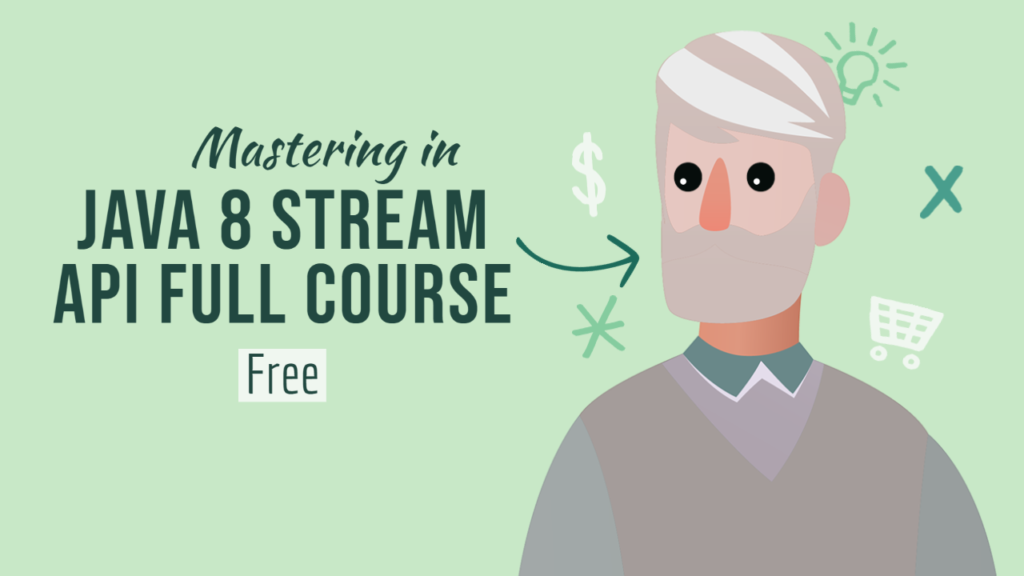## Mastering in Java 8 Stream API Full Course FreeThe Java Stream API, introduced since Java 8, simplifies code logic and reduces lines of code for programming tasks. It works with data stream flow, allowing for the addition of operations to the stream, reducing the number of lines of code.

The Stream API is designed in line with functional programming, implementing program logic by composing functions and executing them in a data flow.

Spring JPA repository is provided for each entity, so that you can use a repository method such as findAll() to fetch records of product, order and customer.

I prepared a Spring Boot project with data model, repositories and sample data loaded into in-memory H2. So, you can play with the stream API using the sample data.

The process is similar to a for-loop, but with simpler and more readable code. To master the Java Stream API, practice 15 exercises based on a data model involving customers, orders, and products.

Each exercise covers a wide range of scenarios, allowing for the use of a Spring JPA repository for each entity. By practicing these exercises, users can gain knowledge on Java Stream API operations and explore other operations.

### Exercise 1 — Obtain a list of products belongs to category “Books” with price > 100

This is obviously a filtering logic; the output should fulfill the two filtering requirements.

So, you can apply 2 filter() operations to obtain the result.

``````List<Product> result = productRepo.findAll()
.stream()
.filter(p -> p.getCategory().equalsIgnoreCase("Books"))
.filter(p -> p.getPrice() > 100)
.collect(Collectors.toList());
``````

### Exercise 2 — Obtain a list of order with products belong to category “Books”

You need to start from the data flow from the order entities and then check if order’s products belong to the category “Books”.

Hence, the filter logic looks into the products stream of each order record and use anyMatch() to determine if any product fulfill the criteria.

``````List<Order> result = orderRepo.findAll()
.stream()
.filter(o ->
o.getProducts()
.stream()
.anyMatch(p -> p.getCategory().equalsIgnoreCase("Baby"))
)
.collect(Collectors.toList());
``````

### Exercise 3 — Obtain a list of product with category = “Toys” and then apply 10% discount

In this exercise, you will see how to transform data using the stream API. After you’ve obtained a product list with a category that belongs to “Toys” using filter(), you can then apply a 10% discount to the product price by using map().

``````List<Product> result = productRepo.findAll()
.stream()
.filter(p -> p.getCategory().equalsIgnoreCase("Toys"))
.map(p -> p.withPrice(p.getPrice() * 0.9))
.collect(Collectors.toList());
``````

### Exercise 4 — Obtain a list of products ordered by customer of tier 2 between 01-Feb-2021 and 01-Apr-2021

This exercise illustrates the usage of flatMap(). You can firstly start from an order list and then filter the list by customer tier and order date. Next, get the product list from each order record and use flatMap() to emit product records into the stream.

For example, if we have 3 order records and each order contains 10 products, then flatMap() will emit 10 data elements for each order record, resulting in 30 (3 x 10) product record output in the stream.

Since product list would contain duplicated product records if multiple orders would include the same product. In order to generate a unique product list, applying distinct() operation can help to produce the unique list.

``````List<Product> result = orderRepo.findAll()
.stream()
.filter(o -> o.getCustomer().getTier() == 2)
.filter(o -> o.getOrderDate().compareTo(LocalDate.of(2021, 2, 1)) >= 0)
.filter(o -> o.getOrderDate().compareTo(LocalDate.of(2021, 4, 1)) <= 0)
.flatMap(o -> o.getProducts().stream())
.distinct()
.collect(Collectors.toList());
``````

### Exercise 5 — Get the cheapest products of “Books” category

One of the effective ways to obtain the product with the lowest price is to sort the product list by price in an ascending order and get the first element.

Java Stream API provides sorted() operation for stream data sorting based on specific field attributes. In order to obtain the first element in the stream, you can use the terminal operation findFirst().

The operation returns the first data element wrapped by Optional as it is possible that the output stream can be empty.

This solution can only return a single product record with the lowest price. If there are multiple product records with the same lowest price, the solution should be modified such that it looks for the lowest price amount first and then filters product records by the price amount so as to get a list of products with the same lowest price.

``````Optional<Product> result = productRepo.findAll()
.stream()
.filter(p -> p.getCategory().equalsIgnoreCase("Books"))
.sorted(Comparator.comparing(Product::getPrice))
.findFirst();
``````

use min() is a better solution as the code is cleaner and it can be done using 2 operatorsn(filter →min) instead of 3 (filter → sorted →findFirst).

``````Optional<Product> result = productRepo.findAll()
.stream()
.filter(p -> p.getCategory().equalsIgnoreCase("Books"))
.min(Comparator.comparing(Product::getPrice));``````

Please stay tuned for Part 2.#### By Tell Me How

It is a technology blog and admin has excellent experience in programming from 5+ year. You can contact us at ceo.tellmehow@gmail.com

#### 6 Incredible Ways Technology is Transforming the Service